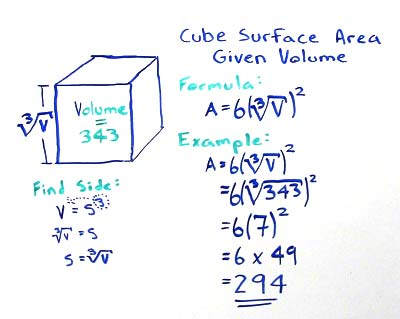# Volume of Cube

## Surface Area using Volume Calculator

A cube with a volume (V) of 125 cubic units has a surface area (A) of 150 square units.

## Cube Surface Area from Volume Formula

If you have a cube with a known volume V and are trying to find the surface area A first calculate the side length S by using this formula:

• S = ∛V

Here is the regular formula for finding the surface area (A) of a cube:

• V = 6S2

You can either find the side length (S) using the very first formula or substitute that formula in to the regular formula for surface area. Here's what the substituted formula would look like:

• V = 6(∛V)2

In this example find the surface area of a cube that have a volume of 343 cubic units. Using the formula above substitute 343 for the cube volume:

• V = 6(∛343)2

The first step is to find the cube root of the volume (343):

• V = 6 x 72

The cube root of 343 is 7. 7 is the side length of the cube. Now square the side length of 7:

• V = 6 x 49

49 is the square area of a single cube face. A cube has 6 faces in total so finish the calculation by multiplying the area of the single face by the number of faces:

• V = 294Given a cube with a volume V the surface area can be found by taking the cube root of the volume to get side length S. The side length S can be used in the normal way to find the surface area of a cube A. Square the side length to find the area of a single face (the area of a square), then multiple by the total number of faces, which will always be 6.

### Formulas

What is the formula for the volume of a Cube?

V = S3

What is the formula for the volume of a Rectangular Prism?

V = L x W x H

### Other Formulae

for cubes with side length S

Cube Surface Area

6S2
Cube Face Diagonal
(√2)S
Cube Space Diagonal
(√3)S
S/2
All formulae...